# Gradient Descent in Linear Regression

In linear regression, the model targets to get the best-fit regression line to predict the value of y based on the given input value (x). While training the model, the model calculates the cost function which measures the Root Mean Squared error between the predicted value (pred) and true value (y). The model targets to minimize the cost function.
To minimize the cost function, the model needs to have the best value of θ1 and θ2. Initially model selects θ1 and θ2 values randomly and then itertively update these value in order to minimize the cost function untill it reaches the minimum. By the time model achieves the minimum cost function, it will have the best θ1 and θ2 values. Using these finally updated values of θ1 and θ2 in the hypothesis equation of linear equation, model predicts the value of x in the best manner it can.
Therefore, the question arises – How θ1 and θ2 values get updated ?
Linear Regression Cost Function: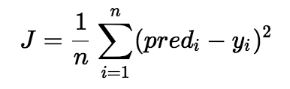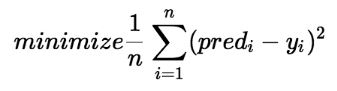Gradient Descent Algorithm For Linear Regression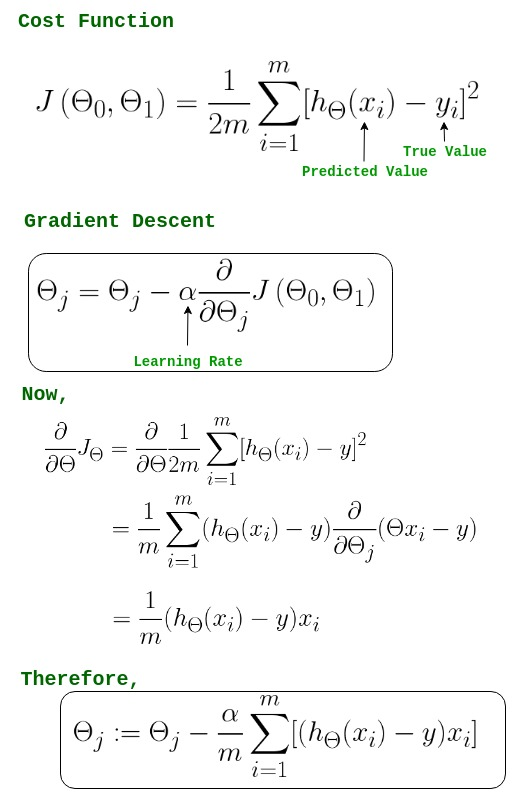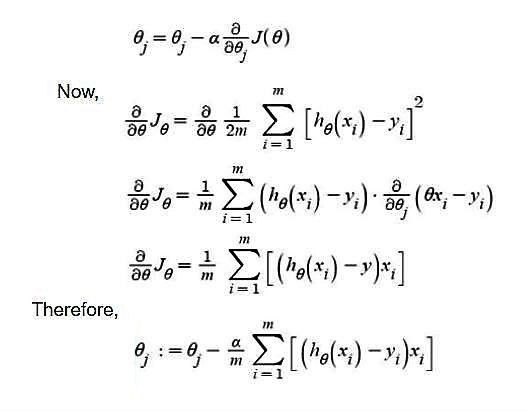```-> θj     : Weights of the hypothesis.
-> hθ(xi) : predicted y value for ith input.
-> j     : Feature index number (can be 0, 1, 2, ......, n).
-> α     : Learning Rate of Gradient Descent.
```

We graph cost function as a function of parameter estimates i.e. parameter range of our hypothesis function and the cost resulting from selecting a particular set of parameters. We move downward towards pits in the graph, to find the minimum value. Way to do this is taking derivative of cost function as explained in the above figure. Gradient Descent step downs the cost function in the direction of the steepest descent. Size of each step is determined by parameter α known as Learning Rate.

In the Gradient Descent algorithm, one can infer two points :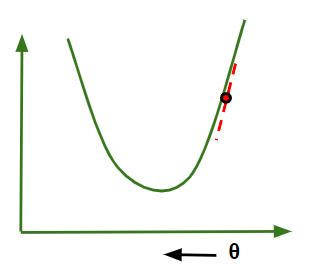1. If slope is +ve : θj = θj – (+ve value). Hence value of θj decreases.
2.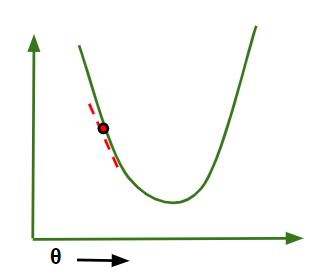3. If slope is -ve : θj = θj – (-ve value). Hence value of θj increases.

The choice of correct learning rate is very important as it ensures that Gradient Descent converges in a reasonable time. :

1. If we choose α to be very large, Gradient Descent can overshoot the minimum. It may fail to converge or even diverge.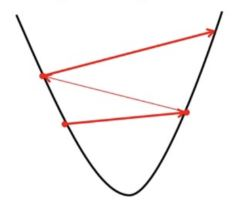2. If we choose α to be very small, Gradient Descent will take small steps to reach local minima and will take a longer time to reach minima.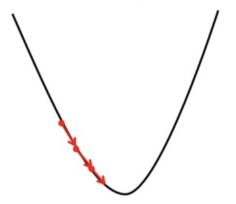3. For linear regression Cost Function graph is always convex shaped.

 `# Implementation of gradient descent in linear regression ` `import` `numpy as np  ` `import` `matplotlib.pyplot as plt  ` ` `  `class` `Linear_Regression: ` `    ``def` `__init__(``self``, X, Y): ` `        ``self``.X ``=` `X ` `        ``self``.Y ``=` `Y ` `        ``self``.b ``=` `[``0``, ``0``] ` `     `  `    ``def` `update_coeffs(``self``, learning_rate): ` `        ``Y_pred ``=` `self``.predict() ` `        ``Y ``=` `self``.Y ` `        ``m ``=` `len``(Y) ` `        ``self``.b[``0``] ``=` `self``.b[``0``] ``-` `(learning_rate ``*` `((``1``/``m) ``*`  `                                  ``np.``sum``(Y_pred ``-` `Y))) ` ` `  `        ``self``.b[``1``] ``=` `self``.b[``1``] ``-` `(learning_rate ``*` `((``1``/``m) ``*`  `                                  ``np.``sum``((Y_pred ``-` `Y) ``*` `self``.X))) ` ` `  `    ``def` `predict(``self``, X``=``[]): ` `        ``Y_pred ``=` `np.array([]) ` `        ``if` `not` `X: X ``=` `self``.X ` `        ``b ``=` `self``.b ` `        ``for` `x ``in` `X: ` `            ``Y_pred ``=` `np.append(Y_pred, b[``0``] ``+` `(b[``1``] ``*` `x)) ` ` `  `        ``return` `Y_pred ` `     `  `    ``def` `get_current_accuracy(``self``, Y_pred): ` `        ``p, e ``=` `Y_pred, ``self``.Y ` `        ``n ``=` `len``(Y_pred) ` `        ``return` `1``-``sum``( ` `            ``[ ` `                ``abs``(p[i]``-``e[i])``/``e[i] ` `                ``for` `i ``in` `range``(n) ` `                ``if` `e[i] !``=` `0``] ` `        ``)``/``n ` `    ``#def predict(self, b, yi): ` ` `  `    ``def` `compute_cost(``self``, Y_pred): ` `        ``m ``=` `len``(``self``.Y) ` `        ``J ``=` `(``1` `/` `2``*``m) ``*` `(np.``sum``(Y_pred ``-` `self``.Y)``*``*``2``) ` `        ``return` `J ` ` `  `    ``def` `plot_best_fit(``self``, Y_pred, fig): ` `                ``f ``=` `plt.figure(fig) ` `                ``plt.scatter(``self``.X, ``self``.Y, color``=``'b'``) ` `                ``plt.plot(``self``.X, Y_pred, color``=``'g'``) ` `                ``f.show() ` ` `  ` `  `def` `main(): ` `    ``X ``=` `np.array([i ``for` `i ``in` `range``(``11``)]) ` `    ``Y ``=` `np.array([``2``*``i ``for` `i ``in` `range``(``11``)]) ` ` `  `    ``regressor ``=` `Linear_Regression(X, Y) ` ` `  `    ``iterations ``=` `0` `    ``steps ``=` `100` `    ``learning_rate ``=` `0.01` `    ``costs ``=` `[] ` `     `  `    ``#original best-fit line ` `    ``Y_pred ``=` `regressor.predict() ` `    ``regressor.plot_best_fit(Y_pred, ``'Initial Best Fit Line'``) ` `     `  ` `  `    ``while` `1``: ` `        ``Y_pred ``=` `regressor.predict() ` `        ``cost ``=` `regressor.compute_cost(Y_pred) ` `        ``costs.append(cost) ` `        ``regressor.update_coeffs(learning_rate) ` `         `  `        ``iterations ``+``=` `1` `        ``if` `iterations ``%` `steps ``=``=` `0``: ` `            ``print``(iterations, ``"epochs elapsed"``) ` `            ``print``(``"Current accuracy is :"``,  ` `                   ``regressor.get_current_accuracy(Y_pred)) ` ` `  `            ``stop ``=` `input``(``"Do you want to stop (y/*)??"``) ` `            ``if` `stop ``=``=` `"y"``: ` `                ``break` ` `  `    ``#final best-fit line ` `    ``regressor.plot_best_fit(Y_pred, ``'Final Best Fit Line'``) ` ` `  `    ``#plot to verify cost fuction decreases ` `    ``h ``=` `plt.figure(``'Verification'``) ` `    ``plt.plot(``range``(iterations), costs, color``=``'b'``) ` `    ``h.show() ` ` `  `    ``# if user wants to predict using the regressor: ` `    ``regressor.predict([i ``for` `i ``in` `range``(``10``)]) ` ` `  `if` `__name__ ``=``=` `'__main__'``: ` `    ``main() `

Output: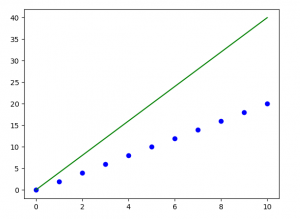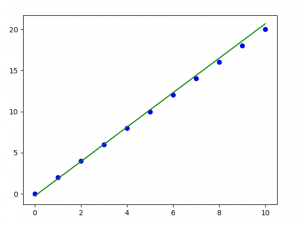Note: Gradient descent sometimes is also implemented using Regularization.

My Personal Notes arrow_drop_upIf you like GeeksforGeeks and would like to contribute, you can also write an article using contribute.geeksforgeeks.org or mail your article to contribute@geeksforgeeks.org. See your article appearing on the GeeksforGeeks main page and help other Geeks.

Please Improve this article if you find anything incorrect by clicking on the "Improve Article" button below.

Improved By : BhushanBorole

Article Tags :
Practice Tags :

3

Please write to us at contribute@geeksforgeeks.org to report any issue with the above content.